# Plus Two Maths Chapter Wise Questions and Answers Chapter 8 Application of Integrals

Students can Download Chapter 8 Application of Integrals Questions and Answers, Plus Two Maths Chapter Wise Questions and Answers helps you to revise the complete Kerala State Syllabus and score more marks in your examinations.

## Kerala Plus Two Maths Chapter Wise Questions and Answers Chapter 8 Application of Integrals

### Plus Two Maths Application of Integrals Four Mark Questions and Answers

Question 1.
Consider the following figure.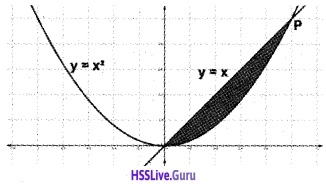1. Find the point of intersection (P) of the given parabola and the line. (2)
2. Find the area of the shaded region. (2)

1. We have, y = x2 and y = x ⇒ x = x2
⇒ x2 – x = 0 ⇒ x(x – 1) = 0 ⇒ x = 0, 1
When x = 0, y =0 and x = 1, y = 1.
Therefore the points of intersections are (0, 0) and(1, 1).

2. Required area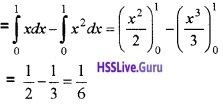Question 2.
1. Find the point of intersection ‘p’ of the given parabola and the line. (2)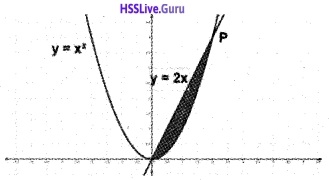2. Find the area of the shaded region. (2)
1. Given, y = x2, y = 2x
⇒ 2x = x2 ⇒ x2 – 2x = 0 ⇒ x(x – 2) = 0 ⇒ x = 0, 2
We have, y = 2x
⇒ when x = 0 ⇒ y = 0, when x = 2 ⇒ y = 4
‘P’ has co-ordinate (2, 4)

2. Area = $$\int_{0}^{2} 2 x d x-\int_{0}^{2} x^{2} d x=\left(x^{2}\right)^{2}-\left(\frac{x^{3}}{3}\right)_{0}^{2}=4-\frac{8}{3}=\frac{12-8}{3}=\frac{4}{3}$$.Question 3.
Consider the following figure.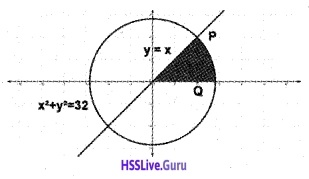1. Find the point of Intersection P of the circle x2 + y2 = 32 and the line y = x. (1)
2. Find the area of the shaded region. (3)

1. x2 + x2 = 32 ⇒ 2x2 = 32 ⇒ x2 = 16 = 4
Therefore the point of intersection P is (4, 4).

2. We have x2 + y2 = 32 ⇒ y = $$\sqrt{32-x^{2}}$$.
The required area =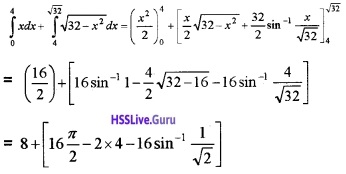= 8 + [8π – 8 – 4π] = 4π.

Question 4.

1. Shade the area enclosed by x2 = 4y, y = 2, y = 4 and the y-axis in the first quadrant. (2)
2. Find the area of the region bound by x2 = 4y, y = 2, y = 4 and the y-axis in the first quadrant. (2)

1.2. AreaQuestion 5.

1. Draw a rough sketch of the graph of the function y2 = 4x. (2)
2. Find the area by the curve and the line x= 2. (2)

1.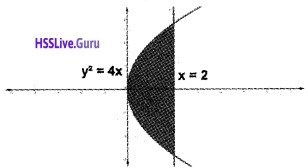2. Area### Plus Two Maths Application of Integrals Six Mark Questions and Answers

Question 1.

1. Draw the graph of y2 = 4x and y = x. (2)
2. Find the points of intersection of y2 = 4x and y = x. (2)
3. Find the area bounded by the graphs.(2)

1. y2 = 4x is a parabola and y = x is a straight line passing through the origin.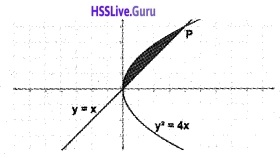2. x2 = 4x ⇒ x2 – 4x = 0 ⇒ x(x – 4) = 0 ⇒ x = 0, 4
When x = 0, y = 0 and when x = 4, y =4. Therefore the points of intersection are (0, 0) and (4, 4).

3. Area bounded by the graphs = Area under the parabola in the first quadrant – Area under the line.Question 2.

1. Draw the graph of the function y = x2 and x = y2 in a coordinate axes. (2)
2. Find the point of intersection of the above graphs. (2)
3. Find the area of the region bounded by the above two curves. (2)

1. The two function are parabolas as shown in the figure.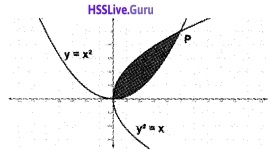2. We have, y = x2 and x = y2
x = (x2)2 ⇒ x – x4 = 0 ⇒ x(1 – x3) = 0 ⇒ x = 0, 1
When x= 1, y= 1 and x = 0, y = 0.
Therefore the point is (0, 0) and (1, 1).

3. The required area = $$\int_{0}^{1} \sqrt{x} d x-\int_{0}^{1} x^{2} d x$$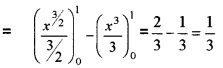Question 3.
Using the figure answer the following questions1. Find the area of the shaded region as the sum of the area of two triangles. (2)
2. Define the function of the given graph. (2)
3. Verify the area of the shaded region using integration. (2)

1. Area = Area of ∆OAD + Area of ∆ ABC
$$\frac{1}{2}$$ × 3 × 3 + $$\frac{1}{2}$$ × 2 × 2 = $$\frac{9}{2}$$ + 2 = $$\frac{13}{2}$$.

2. Area = $$\int_{0}^{5}$$f(x) dx = $$\int_{0}^{3}$$(-x + 3) dx + $$\int_{3}^{5}$$(x – 5) dxTherefore verified.Question 4.
The figure given below contains a straight line L with slope $$\sqrt{8}$$ and a circle.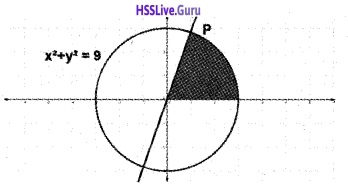1. Find the equation of the line L and circle. (1)
2. Find the point of intersection P. (2)
3. Find the area of the shaded region. (3)

1. The line L passes through origin and have slope 3, therefore its equation is y = $$\sqrt{8}$$ x. The circle passes through origin and have radius 3, therefore its equation is x2 + y2 = 9.

2. We have, y =3x and x2 + y2 = 9
⇒ x2 + ($$\sqrt{8}$$x)2 = 9 ⇒ 9x2 = 9
⇒ x = 1
∴ y = $$\sqrt{8}$$ × 1 = $$\sqrt{8}$$.
Therefore, coordinate of ‘P’ is (1, $$\sqrt{8}$$).

3. Area of the shaded regionQuestion 5.
Using the given figure answer the following1. Define the equation of the circle and ellipse in the figure. (1)
2. Find the area of the ellipse using integration. (4)
3. Find the area of the shaded region. (Use formula to find the area of the circle.) (1)

1. From the figure equation of the circle is x2 + y2 = 4 and that of the ellipse is $$\frac{x^{2}}{4}+\frac{y^{2}}{1}=1$$.

2. We have, $$\frac{x^{2}}{4}+\frac{y^{2}}{1}=1$$
⇒ y2 = 1 ⇒ y = $$\frac{1}{2} \sqrt{4-x^{2}}$$
Area of the ellipse = 4 $$\int_{0}^{2}$$y dx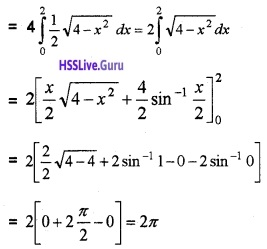3. Area of the circle of radius 2 = π (2)2 = 4π
∴ Area of the shaded region = Area of the circle – Area of the ellipse
= 4π – 2π = 2π.

Question 6.

1. Find the area bounded by the curve y = sin x with X – axis, between x = 0 and x = 2π. (2)
2. Find the area of the region bounded by the curve y = x2 and y = |x| (4)

1. Area of y = sin x in each loop is same. Therefore;
2$$\int_{0}^{\pi}$$sin xdx = $$-2(\cos x)_{0}^{\pi}$$ = -2 (cos π – cos0)
= -2(-1 – 1) = 4

2.Area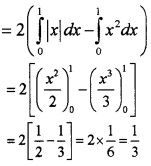Question 7.
Using integration, find the area of the region bounded by the triangle whose vertices are(-1, 1), (0, 5) and (3, 2). (6)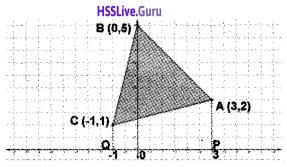Equation of BC is $$\frac{y-5}{1-5}=\frac{x-0}{-1-0}$$
⇒ y – 5 = 4x ⇒ 4x – y + 5 = 0 ⇒ y = 4x + 5
Equation of AB is x + y – 5 = 0 ⇒ y = 5 – x
Equation of AC is x – 4y + 5 = 0 ⇒ y = $$\frac{x}{4}+\frac{5}{2}$$
The required area = Area of the region PABCQP – Area of the region PACQP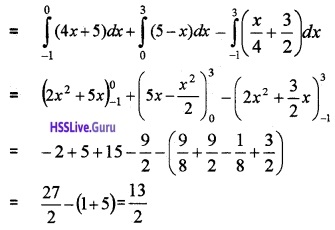Question 8.
Consider the functions f(x) = sin x and g(x) = cosx in the interval [0, 2π]

1. Find the x coordinates of the meeting points of the functions. (1)
2. Draw the rough sketch of the above functions. (2)
3. Find the area enclosed by these curves in the given interval. (3)

1. f(x) = sin x and g(x) = cos x meet at multiples of $$\frac{\pi}{4}$$
x = $$\frac{\pi}{4}$$, $$\frac{5 \pi}{4}$$.

2.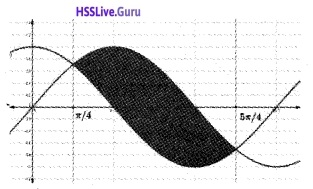3. Area = 2{Area under f(x) = sinx from $$\frac{\pi}{4}$$ to π – Area under g(x) = cosx from $$\frac{\pi}{4}$$ to $$\frac{\pi}{2}$$}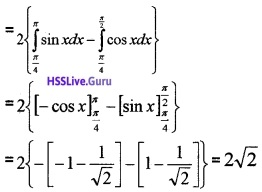Question 9.

Evaluate $$\int_{0}^{r} \sqrt{r^{2}-x^{2}} d x$$, where r is a fixed positive number. Hence prove the area of the circle of radius r is π r2. (2)
Find the area of the circle, x2 + y2 = 16 which is exterior to parabola y2 = 6x. (4)

1.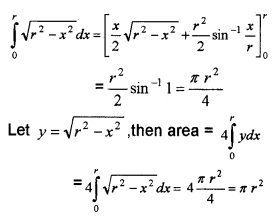2. Given, x2 + y2 = 16 and y2 = 6x ⇒ x2 + 6x = 16 ⇒ x2 + 6x – 16 = 0 ⇒ (x + 8)(x – 2) = 0 ⇒ x = -8, 2.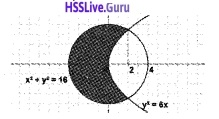Area = Area of the circle – Interior area of the parabola.Question 10.
Using the figure answer the following questions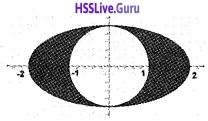1. Define the equation of the ellipse and circle in the given figure. (1)
2. Find the area of the ellipse using integration. (4)
3. Find the area of the shaded region. (Area of the circle can be found using direct formula). (1)

1. Equation of the ellipse is $$\frac{x^{2}}{4}+\frac{y^{2}}{1}=1$$ and circle is x2 + y2 = 1.
2. We have, $$\frac{x^{2}}{4}+\frac{y^{2}}{1}=1$$
⇒ y2 = 1 ⇒ y = $$\frac{1}{2} \sqrt{4-x^{2}}$$
Area of the ellipse = 4 $$\int_{0}^{2}$$y dx